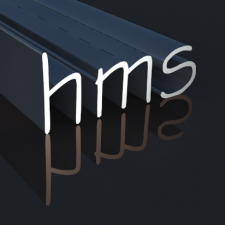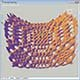# Add Sphere to Centroid of 3D Solids via LISP## Recommended PostsHi everyone, here's what I'm trying to do:

I have a lot of curved bridge girders, and I am using MASSPROP to find the centroid location, and then manually typing in the centroid coordinates as the center point of a sphere.

Awhile back, I found this code that is supposed to do precisely that, but these days it is adding a sphere at a corner point, not the centroid.

Any help would be greatly appreciated!

```(defun c:masscent(/ sset1 slng1 cnt1 cnt2 clist sent1 xlist ylist zlist xpt ypt zpt cpt rad vol1 vlist ucp)

(vl-cmdf "._undo" "_end")
(vl-cmdf "._undo" "_group")
(vl-cmdf "._ucs" "_w")
(prompt "\nSelect solids for centroid location. ")
(setq sset1 (ssget)
slng1 (sslength sset1)
cnt1 0
cnt2 0
clist nil
)

(repeat slng1
(setq sent1 (vlax-ename->vla-object (ssname sset1 cnt2)))
(if (vlax-property-available-p sent1 "Centroid")
(progn
(setq clist (append clist (list (vlax-get sent1 "Centroid"))))
(setq vlist (append vlist (list (vlax-get sent1 "Volume"))))
(setq cnt1 (1+ cnt1))
)
)
(Setq cnt2 (1+ cnt2))
)
(setq xlist (mapcar 'car clist))
(setq xpt (car xlist))
(setq ypt (car ylist))
(Setq zpt (car zlist))
(setq vol1 (car vlist))
(repeat (fix (- (vl-list-length clist) 1))
(setq xlist (cdr xlist))
(setq xpt (+ xpt (car xlist)))
(setq ylist (cdr ylist))
(setq ypt (+ ypt (car ylist)))
(setq zlist (cdr zlist))
(setq zpt (+ zpt (car zlist)))
(setq vlist (cdr vlist))
(Setq vol1 (+ vol1 (car vlist)))
)
(setq cpt (list xpt ypt zpt))
(setq cpt (mapcar '(lambda (x) (/ x cnt1)) cpt))
(setq vol1 (strcat (rtos vol1 2 5) " cu. in."))
(vl-cmdf "._ucs" "_p")
(vl-cmdf "._undo" "_end")
(princ "\n")
(princ "Centroid point: ")
(princ cpt)
(princ (strcat " - Volume: " vol1))
(princ)
)```

##### Share on other sites```(if clist(vl-cmdf "._sphere" clist rad)

##### Share on other sitesparkerdepriest,

if only need to add spheres to centroid of 3D solids, try this :

```(defun c:test (/ ss rad itm obj cpt)
(prompt "\nSelect solids for centroid location. ")
(if (setq ss (ssget "_:L" '((0 . "3DSOLID"))))
(progn
itm 0
)
(repeat (sslength ss)
(setq obj (vlax-ename->vla-object (ssname ss itm))
cpt (vlax-safearray->list
(vlax-variant-value (vlax-get-property obj 'Centroid))
)
)
(vl-cmdf "._sphere" "_Non" (trans cpt 0 1) rad)
(setq itm (1+ itm))
);; repeat
);; progn
);; if
(princ)
)
```

tested minimally,

Henrique

##### Share on other sitesHenrique , See the difference between this code and yours with no offense .

```(defun c:test (/ ss rad itm obj cpt) (vl-load-com)
(prompt "\nSelect solids for centroid location. ")
(if (and (setq ss (ssget "_:L" '((0 . "3DSOLID"))))
)
(repeat (setq itm (sslength ss))
(setq  obj (vlax-ename->vla-object (ssname ss (setq itm (1- itm)))))
(if (vlax-property-available-p obj 'Centroid)
(progn
(setq cpt (vlax-get obj 'Centroid))
(vl-cmdf "._sphere" "_Non" (trans cpt 0 1) rad)
)))
)
(princ)
)
```

##### Share on other sitesThe Centroid property is expressed relative to the UCS, not WCS.

##### Share on other sitesTharwat

no offense at all,

better organized and I, as always,

forgot to test if the property is available ...

"Many years without coding"

Cheers

Henrique

##### Share on other sitesThe Centroid property is expressed relative to the UCS, not WCS.

Hi Lee, I'm confused here.

The Centroid property seems to be referred to WCS, not to UCS as this expressions seem to show:

_\$ (setq sph (vlax-ename->vla-object (entlast)))

#

_\$ (vlax-safearray->list (vlax-variant-value (vla-get-centroid sph)))

(0.0 0.0 0.0)

Now I change the UCS, displacing its origin and rotating the axes.

And this is the result:

_\$ (vlax-safearray->list (vlax-variant-value (vla-get-centroid sph)))

(0.0 0.0 0.0)

As to the CENTER argument if using the AddSphere method, which I would recommend instead of the command, it is according to the documentation, the 3D WCS coordinates specifying the sphere center point.

Of course, using command you should specify the center argument as UCS, but it should not be confused with the CENTROID property.

Best wishes for 2013!

##### Share on other sitesMy apologies, it would appear that the Centroid property is expressed relative to WCS for 3DSolids and expressed relative to UCS for Regions. Of course, where the Sphere is concered, the centroid = center in any case.

##### Share on other sites

• 1 year later...i have one question, being a rookie at this, can someone explain the end of the 7th line of code to me..

`(setq  obj (vlax-ename->vla-object (ssname ss [b][u](setq itm (1- itm))[/u][/b])))`

having trouble understanding this.

isn't the index for ssname going to be negative (not allowed) with this ?

obviously not, it's working but stil i have to ask..

can this be updated to work on finding a unified center of mass for multiple objects ?

thanks guys

##### Share on other sitesDecrementing variable itm is processed only (sslength ss) number of times, so itm never reaches negative value...

```(repeat (setq itm (sslength ss))
```

As for unified center of mass, I suggest that you use "UNION" command and apply it on all 3DSOLID entities and then obtain unified centroid coordinates... After that go one step "UNDO" to restore unified 3DSOLID to separate 3DSOLID entities...

##### Share on other sitesAs for unified center of mass, I suggest that you use "UNION" command and apply it on all 3DSOLID entities and then obtain unified centroid coordinates... After that go one step "UNDO" to restore unified 3DSOLID to separate 3DSOLID entities...

Not necessary. You can calculate the center of mass as weighted average:

Xg = (sum Xi*Vi) / (sum Vi); idem for Yg and Zg.

```(defun c:test ( / ss v c l p i)
(if
(setq ss (ssget '((0 . "3DSOLID"))))
(progn
(repeat (setq i (sslength ss))
(setq o (vlax-ename->vla-object (ssname ss (setq i (1- i))))
c (cons (vlax-get o 'centroid) c)
v (cons (vlax-get o 'volume)   v)
)
)
(setq l (mapcar '(lambda (a b) (mapcar '* a (list b b b))) c v)
v (apply '+ v)
p (mapcar '/ (apply 'mapcar (cons '+ l)) (list v v v))
)
(entmake
(list
'(0 . "POINT")
(cons 10 p)
)
)
)
)
(princ)
)```

##### Share on other sitesNot necessary. You can calculate the center of mass as weighted average:

Xg = (sum Xi*Vi) / (sum Vi); idem for Yg and Zg.

```(defun c:test ( / ss v c l p i)
(if
(setq ss (ssget '((0 . "3DSOLID"))))
(progn
(repeat (setq i (sslength ss))
(setq o (vlax-ename->vla-object (ssname ss (setq i (1- i))))
c (cons (vlax-get o 'centroid) c)
v (cons (vlax-get o 'volume)   v)
)
)
(setq l (mapcar '(lambda (a b) (mapcar '* a (list b b b))) c v)
v (apply '+ v)
p (mapcar '/ (apply 'mapcar (cons '+ l)) (list v v v))
)
(entmake
(list
'(0 . "POINT")
(cons 10 p)
)
)
)
)
(princ)
)```

I don't agree with you Stefan... Try to make one SPHERE and one BOX that interfere each other... Apply your code, type ID on your point, and then do UNION, select both 3DSOLIDs and type MASSPROP... Look for Centroid coordinates - you'll see that they differs slightly, but it's different...

##### Share on other sitesYou're right. There are two different things, with two different results. It's up to OP to chose the right one.

##### Share on other sitesthanks marko,

after some time of the screen and refreshing the lisp reference guide a couple of times i saw where i was wrong..

i mistaked the decrementing operation for a subtitution hastingly.. i saw 1- itm and thought (one minus itm=definitely less than zero)..

didn't know the syntax..

anyways, thanks a lot guys, will check the unified COG problem now..

cheers.

## Join the conversation

You can post now and register later. If you have an account, sign in now to post with your account.
Note: Your post will require moderator approval before it will be visible.Unfortunately, your content contains terms that we do not allow. Please edit your content to remove the highlighted words below.

×   Pasted as rich text.   Restore formatting

Only 75 emoji are allowed.